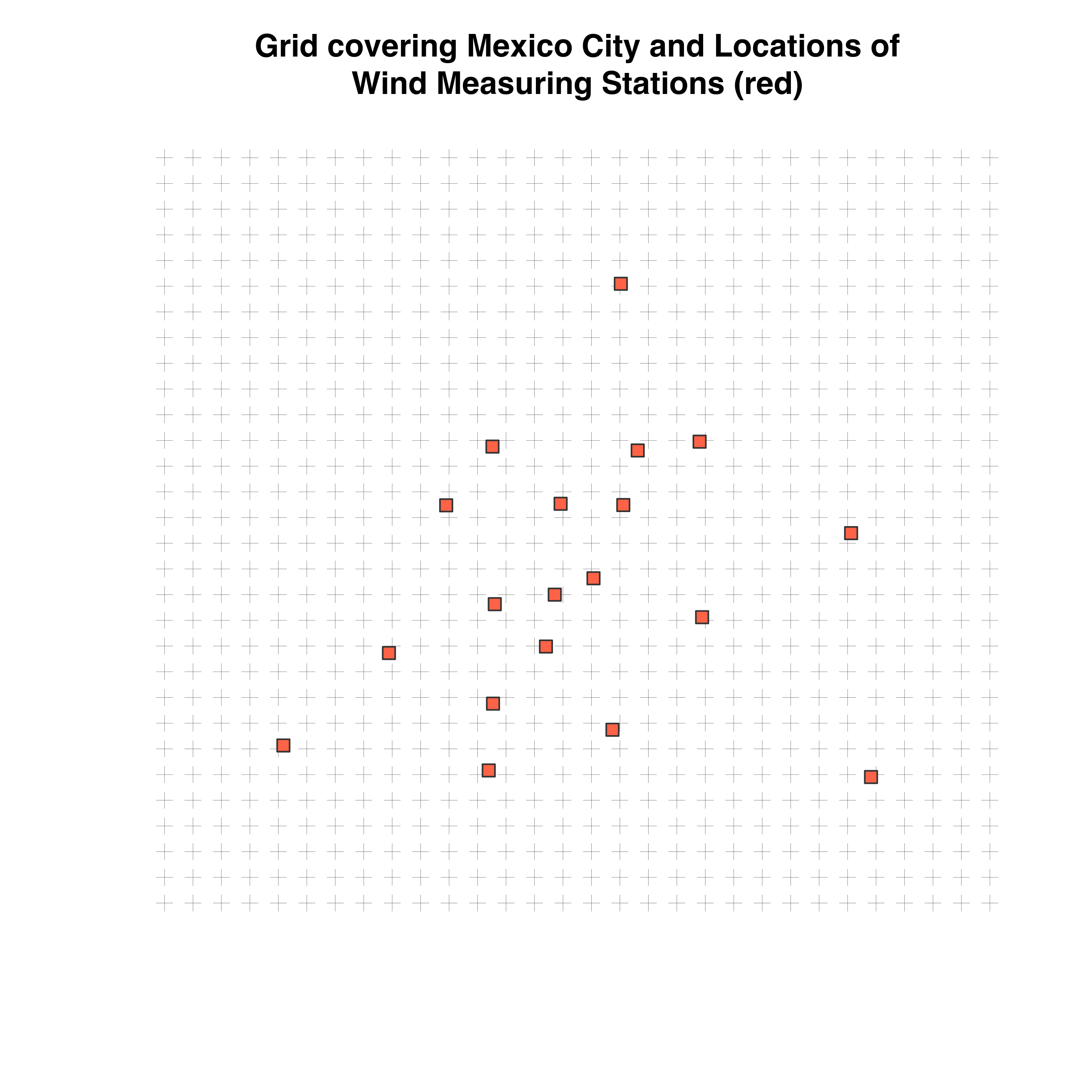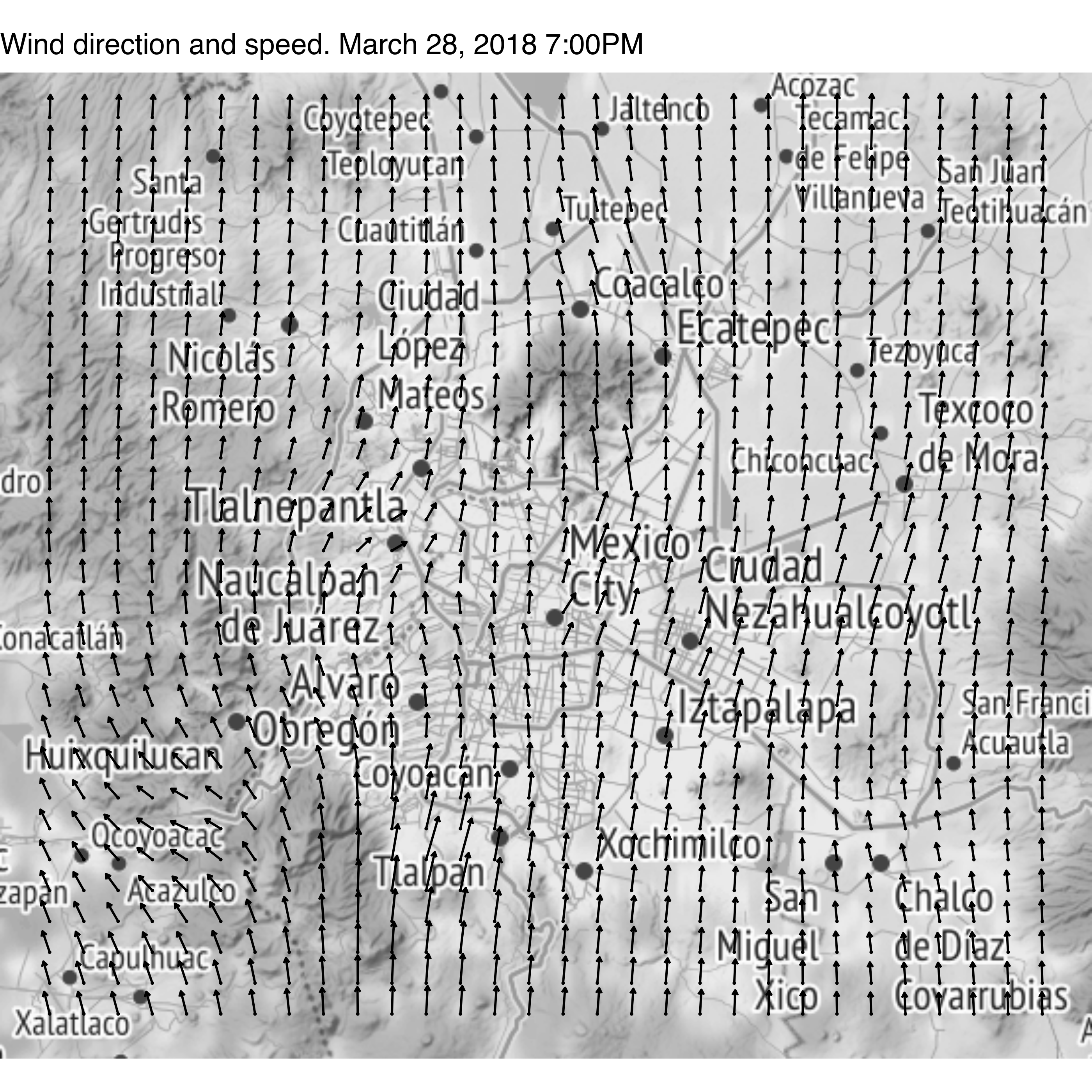We can use the idw360 function to extrapolate wind direction and speed inside a grid. First we load the packages:

## Auto-install required R packages
if (!require("pacman")) install.packages("pacman")
pacman::p_load(aire.zmvm, dplyr, ggplot2, lubridate, stringr, sp, mapproj,
gstat, ggmap)

# download wind speed and direction and filter March 28, 2018 7:00 PM
wsp <- get_station_month_data(criterion = "HORARIOS",
pollutant = "WSP",
2018, 3)%>%
filter(date == "2018-03-28" & hour == 19) %>%
na.omit()
wdr <- get_station_month_data(criterion = "HORARIOS",
pollutant = "WDR",
year = 2018, 3) %>%
filter(date == "2018-03-28" & hour == 19)  %>%
na.omit()

This is what the data look like

knitr::kable(head(wsp))
date hour station_code pollutant unit value
2 2018-03-28 19 AJM WSP m/s 10.2
5 2018-03-28 19 BJU WSP m/s 4.6
9 2018-03-28 19 MON WSP m/s 6.5
10 2018-03-28 19 CHO WSP m/s 3.5
12 2018-03-28 19 CUA WSP m/s 4.3
14 2018-03-28 19 FAC WSP m/s 3.8
knitr::kable(head(wdr))
date hour station_code pollutant unit value
2 2018-03-28 19 AJM WDR ° 198
5 2018-03-28 19 BJU WDR ° 169
9 2018-03-28 19 MON WDR ° 165
10 2018-03-28 19 CHO WDR ° 150
12 2018-03-28 19 CUA WDR ° 246
14 2018-03-28 19 FAC WDR ° 205
We ca n create a po int gri d over which to extrapolate the wi nd values
## Location of sensors. First column x/longitude, second y/latitude
locations <- stations[, c("station_code", "lon", "lat")]
locations <- merge(wdr, locations)
coordinates(locations) <- ~lon+lat
proj4string(locations) <- CRS("+proj=longlat +ellps=WGS84 +no_defs +towgs84=0,0,0")

## The grid for which to extrapolate values
pixels = 30
grid <- expand.grid(lon = seq((min(coordinates(locations)[, 1]) - .1),
(max(coordinates(locations)[, 1]) + .1),
length.out = pixels),
lat = seq((min(coordinates(locations)[, 2]) - .1),
(max(coordinates(locations)[, 2]) + .1),
length.out = pixels))
grid<- SpatialPoints(grid)
proj4string(grid) <- CRS("+proj=longlat +ellps=WGS84 +no_defs +towgs84=0,0,0")

plot(grid, main = "Grid covering Mexico City and Locations of\nWind Measuring Stations (red)",
col = "#666666", lwd = .2)
# Plot the locations of the stations
geog <- stations[stations$station_code %in% unique(wsp$station_code), c("lat", "lon")]
coordinates(geog) <- ~lon+lat
points(geog, pch = 22, col = "#333333", bg = "tomato1", cex = 1.2)extrapolate using inverse distance weighting

# extrapolate wind direction
res <- idw360(wdr$value, locations, grid) # extrapolate wind speed wsp <- left_join(wsp, stations, by = "station_code") wsp <- wsp[!is.na(wsp$value),]
wsp <- wsp[,c("lat", "lon", "value")]
coordinates(wsp) <- ~lon+lat
proj4string(wsp) <- CRS("+proj=longlat +ellps=WGS84 +no_defs +towgs84=0,0,0")
wsp <- idw(value ~ 1, wsp, grid, idp = 2, debug.level = 0)
wsp = as.data.frame(wsp)
names(wsp) <- c("lon", "lat", "var1.wsp", "var1.var")

# Combine wind direction and speed with the grid
df <- cbind(res, as.data.frame(grid), wsp = wsp$var1.wsp) and finally we plot the data (Note that you may have to install a beta version of ggmap by running: devtools::install_github("dkahle/ggmap", ref = "tidyup")) df$group <- 1
qmplot(lon, lat, data = df, geom = "blank",
maptype = "toner-lite", source = "stamen",  zoom = 10, mapcolor = "bw") +
geom_point(size = .1) +
# The wind direction compass starts where the 90 degree mark is located
geom_spoke(aes(angle = ((270 -  pred) %% 360) * pi / 180,
ggtitle("Wind direction and speed. March 28, 2018 7:00PM")